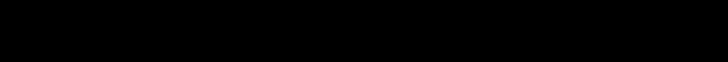Pattern Analysis Laboratory
Department of Mathematics, Colorado State UniversityThe Pattern Analysis Laboratory has been made possible by sustained support from the Department of Mathematics and the College of Natural Sciences at Colorado State University.  We have also received support from other  sources including the National Science Foundation, Air Force Office of Scientific Research, the Defense Advanced Projects Research Agency, Honeywell, IBM, Siemens and the United States Forest Service. Current Funding.
Analysis of High Dimensional Data Sets
The understanding of patterns in high-dimensional data sets is proving to be a key ingredient to knowledge discovery.  The analysis of massive data sets involves a myriad of theoretical, algorithmic and computational challenges from which has emerged a new field of applied mathematics.  The Pattern Analysis Laboratory supports faculty and student research at Colorado State University with an emphasis on mathematical approaches for data analysis.   This research is concerned with the analysis, synthesis and modeling of patterns in data for knowledge discovery.
Mathematics and the Study of Patterns
The analysis of patterns in data has typically been a subject in statistics and engineering.  Recently, however, fundamental mathematical theory in areas such as differential geometry and topology has provided a new mathematical framework and insights for understanding large data sets residing in spaces of large ambient dimensions.  The research at the Pattern Analysis Laboratory emphasizes the transition of mathematical theory to efficient algorithms for exploring, understanding and modeling massive data sets.  It is remarkable how a mathematical framework can find applications across an array of seemingly disparate problems.  Areas of interest include:

Low dimensional embeddings
Representation of data on Grassmann, Stiefel and flag manifolds
Geometric optimization
Computational geometry and topology